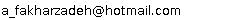••••••••Email:### IPM Positions

Non Resident Researcher (non-resident), School of Mathematics
(2001 - 2002 )

### Past IPM Positions

Associate Researcher (non-resident), School of Mathematics
(2000 - 2001)

### Research Activities

In the previous works, we applied shape-measure method to find the optimal domain (in plan) for control systems governed by elliptic and wave equations with the given conditions. Here we intend to solve an optimal shape design problem in the similar way for diffusion systems involved with the initial, boundary and terminal conditions so that the wished integral performance criteria (defined on the domain and its boundary) be minimized. We remind that Rubio in 1995 solved the similar optimal control problem by embedding method; he also applied the Rudolph approach for getting better approximation.
?

To be sure that the domain is simple in orthogonal coordinates, it is divided into a fixed and a variable curves. Then by idea of approximating a curve with broken lines and fixing the y-components, each admissible domain can be represented with finite number of variables (domain variables). Therefore in the first step of shape-measure method, for a given domain, we will determine the optimal control and the optimal value of objective function by applying the obtained results from Rubio. Then a vector function on the set of admissible domains will be set up in which it gives the optimal valve of objective function for a given domain and its related optimal control function. Afterwards, in the second step, by use of a standard minimization algorithm, the nearly optimal domain and its related optimal control function will be determined at the same time. Numerical examples will be also given.
?

To get a better approximation, for the first time in optimal shape design problems, we may apply the Glashoff-Gustafson approach while Rubio used the Rudolph's one.

### Present Research Project at IPM

Designing an Optimal Domain for a Diffusion Equation by Use of Measures

### Related Papers

 1. Best domain for an elliptic problem in Cartesian coordinates by means of shape-measureAsian Journal of Control 11 (2009), 536-547  [abstract]
 2. A. Fakharzadeh J. Finding the optimum domain of a nonlinear wave optimal control system by measuresJ. Appl. Math. Comput. 13 (2003), 183-194  [abstract]
 [Back]
scroll left or right